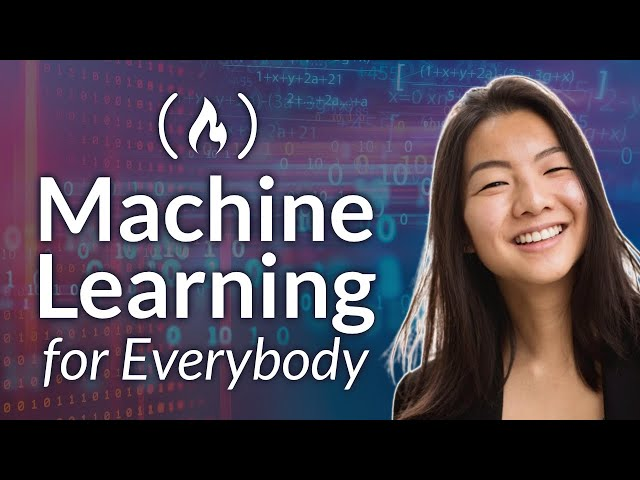# Learn Machine Learning with Google Colab: Predict Radiation Patterns and Cluster Wheat VarietiesTLDR Kylie Ying demonstrates how to use Google Colab to program supervised and unsupervised learning models to predict particle radiation patterns and cluster different varieties of wheat.

### Machine Learning Fundamentals

• 🤖
Kylie Ying teaches machine learning in a way that is accessible to absolute beginners, making it easier for more people to learn about this important field.
• 🧠
In supervised learning, labeled inputs are used to train models and learn outputs of different new inputs that we might feed our model, while in unsupervised learning, unlabeled data is used to learn about patterns in the data by finding some sort of structure in our unlabeled data.
• 📊
Loss is a numerical quantity used to measure the difference between the predicted output and the true value, and it is used to train and evaluate machine learning models.
• 🤖
Using packages like sklearn can save time and prevent coding errors when implementing machine learning algorithms.
• 📈
In SVMs, the goal is not only to separate the two classes well, but also to maximize the margins between the data points and the dividing line, with the data points on the margins being called support vectors.
• 🤖
SVMs are powerful classification models with the potential to achieve high accuracy.
• 📈
Gradient descent can be used to follow the slope of a loss function and adjust weights in a neural net to improve predicted output.
• 🤖
Tensorflow makes it easy to define and train machine learning models, allowing developers to focus on the problem they are trying to solve rather than the technical details of programming the model.
• 🤖
Using a neural net model can provide a non-linear predictor for estimating values, even with multiple inputs.

### Model Evaluation and Optimization

• 📊
Precision and recall scores are important measures in evaluating the performance of a model, with precision measuring the accuracy of positive predictions and recall measuring the ability to correctly identify positive instances.
• 🔍
Grid search can be used to explore different combinations of hyperparameters and their effects on training, potentially improving model performance.
• 📈
Linear regression is a method of finding the line of best fit that will help decrease the measure of error with respect to all the data points in a data set and come up with the best prediction for all of them.
• 🚲
Regression analysis can be used to predict a continuous value, such as the number of bikes rented at each hour in a bike sharing dataset.

## Q&A

• What is supervised learning?

Supervised learning involves predicting discrete classes or continuous values and evaluating the model's performance using data sets.

• How can we measure loss in a model?

Loss is the difference between the predicted and actual label, and it can be measured using l1, l2, and binary cross entropy loss functions.

• What is the purpose of splitting data into train, valid, and test sets?

Splitting data into train, valid, and test sets allows us to train the model, validate its performance, and test it on unseen data.

• How does k-nearest neighbors classification work?

K-nearest neighbors classifies data points by looking at the majority label of the points around them, using a distance function such as Euclidean distance.

• What is the purpose of using TensorFlow in machine learning?

TensorFlow is an open source library that helps develop and train machine learning models, specifically for neural networks with layers of dense nodes and activation functions.

## Timestamped Summary

• 🤖
00:00
Kylie Ying is teaching how to use Google Colab to program supervised and unsupervised learning models to predict particle radiation patterns using the Magic Gamma Telescope data set.
• 🤔
34:50
K-nearest neighbors is a classification algorithm that uses a distance function to classify data points based on their features.
• 🤔
55:04
Using Naive Bayes, linear regression, logistic regression, and SVMs, we can calculate the probability of various outcomes with varying levels of accuracy.
• 🤖
1:36:18
Train and plot a model with TensorFlow to adjust weights and minimize loss for improved output.
• 🤔
2:02:04
Using linear regression to find the line of best fit, we measure the accuracy of the model by calculating the residuals, MAE, MSE, RMSE, RSS, TSS, and r-squared.
• 🤖
2:38:21
Using a neural net model, we can fit a better model to our data set with all the features and plot the loss of the history after training.
• 🤔
3:08:54
Unsupervised learning algorithms such as K-means clustering, Expectation Maximization, and PCA can be used to cluster different varieties of wheat and visualize the results.
• 🤔
3:47:40
K-means clustering and PCA were used to reduce a 7-dimensional data set to a 2-dimensional representation and successfully predict three categories without labels.Report the articleThank you for the feedback